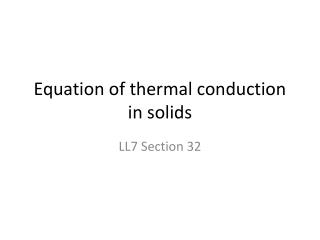DownloadDownload PresentationEquation of thermal conduction in solids

# Equation of thermal conduction in solids

Download Presentation## Equation of thermal conduction in solids

- - - - - - - - - - - - - - - - - - - - - - - - - - - E N D - - - - - - - - - - - - - - - - - - - - - - - - - - -
##### Presentation Transcript

1. Equation of thermal conduction in solids LL7 Section 32

2. Solids

3. Rate of change of heat energy in a volume = negative of heat energy flux q out of volume through the boundary surface = Negative volume integral of div qby Gauss’s theorem.

4. Per unit volume…

5. Because of second term…